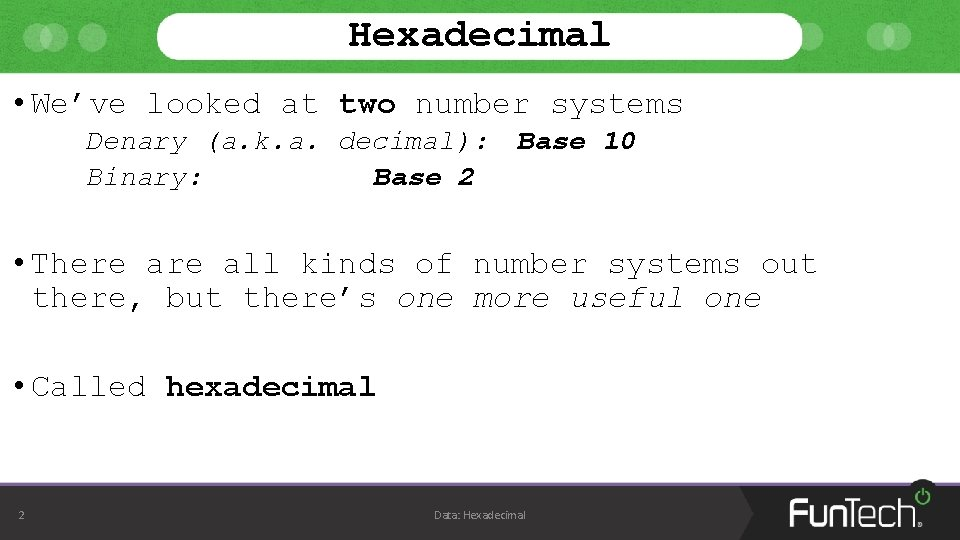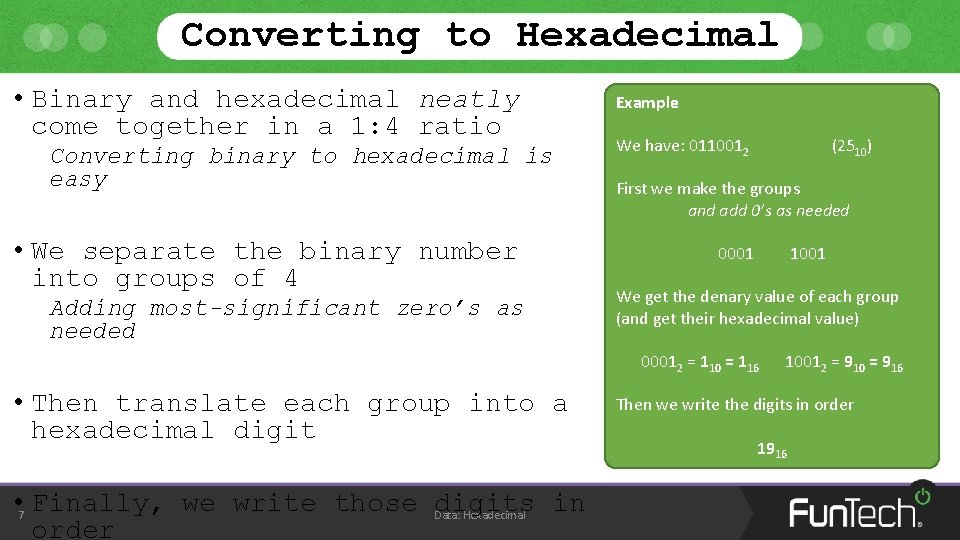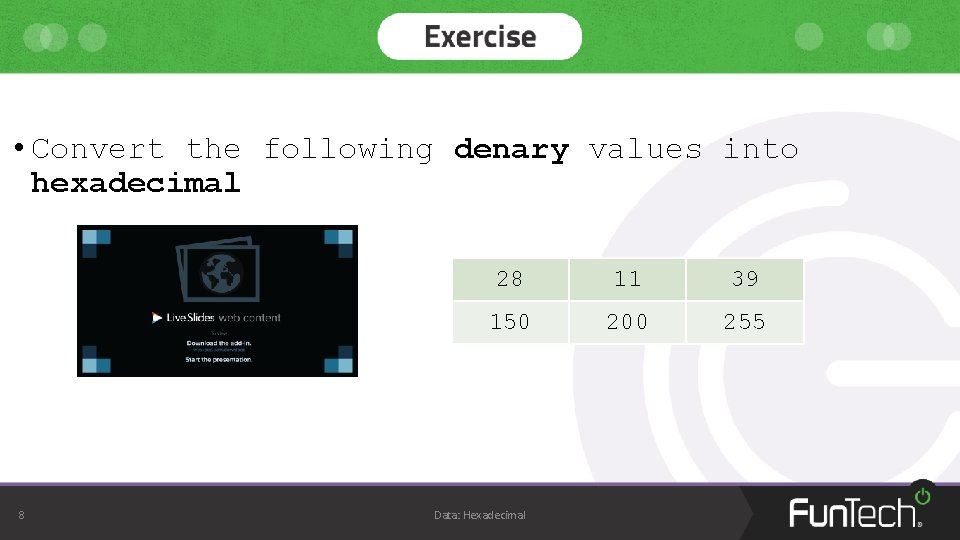• Slides: 12Topic 3: Data Hexadecimal Computer ScienceHexadecimal • We’ve looked at two number systems Denary (a. k. a. decimal): Binary: Base 2 Base 10 • There all kinds of number systems out there, but there’s one more useful one • Called hexadecimal 2 Data: HexadecimalHexadecimal • Hexadecimal is a base 16 number system • First, let’s look at the values for a possible digit: Hexadecim al 0 1 0 -9 2 3 4 5 Denary 0 1 2 3 4 5 then 6 7 8 9 A B 8 9 10 11 A-F C 12 D E F 13 14 15 • There’s a reason why we look at hexadecimal To do with its relation to binary 3 Data: HexadecimalHexadecimal • Let’s look at the same values • But this time we’ll include the binary values Hexadecim al 0 1 2 3 4 5 6 7 8 9 A B C D E F Denary 0 1 2 3 4 5 6 7 8 9 10 11 12 13 14 15 Binary 000 0 000 1 001 0 001 1 010 0 010 1 011 0 011 1 100 0 100 1 101 0 101 1 110 0 110 1 111 0 111 1 • Notice how the denary value 15 fits into 4 binary bits, but 1 hexadecimal digit? 4 Data: HexadecimalHexadecimal Hexadecim al 0 1 2 3 4 5 6 7 8 9 A B C D E F Denary 0 1 2 3 4 5 6 7 8 9 10 11 12 13 14 15 Binary 000 0 000 1 001 0 001 1 010 0 010 1 011 0 011 1 100 0 100 1 101 0 101 1 110 0 110 1 111 0 111 1 • This nice ‘coincidence’ makes representing binary easy Instead of using 4 binary digits, we can use 1 hexadecimal digit • It’s worth noting that this easier representation is purely for us 5 Hexadecimal Computers will still Data: use binaryConverting to Hexadecimal • As we have another number system, we’ll need to know how to convert From denary to hexadecimal binary to hexadecimal to denary hexadecimal to binary • Luckily, the best way to convert between hexadecimal and denary is to go through binary So we only need to work out how to go between hexadecimal and binary Denary 6 Binary Data: HexadecimalConverting to Hexadecimal • Binary and hexadecimal neatly come together in a 1: 4 ratio Converting binary to hexadecimal is easy • We separate the binary number into groups of 4 Adding most-significant zero’s as needed Example We have: 0110012 (2510) First we make the groups and add 0’s as needed 0001 1001 We get the denary value of each group (and get their hexadecimal value) 00012 = 110 = 116 • Then translate each group into a hexadecimal digit • 7 Finally, we write those Data: digits in Hexadecimal order 10012 = 910 = 916 Then we write the digits in order 1916• Convert the following denary values into hexadecimal 8 28 11 39 150 200 255 Data: HexadecimalConverting from Hexadecimal • Converting from hexadecimal to binary works in the exact opposite way Example • We split the hexadecimal number into its digits First we split the number into its digits (and convert each one into denary) • We find the denary value of each digit • We convert that denary value into a 4 -bit binary number • Finally, we write the binary numbers in order 9 From left-to-right Data: Hexadecimal We have: A 516 A 16 = 1010 516 = 510 Then we convert each number into 4 -bit binary 1010 = 10102 510 = 01012 Then we write these numbers on after the other 101001012• Convert the following denary values into hexadecimal 10 F 2 A 1 8 C B 1 CD D 7 Data: HexadecimalSome Uses of Hexadecimal • There are two common uses of hexadecimal Colours Some systems use 1 byte per colour (red, green, and blue) Makes 24 -bits in total Can be shortened to a 6 -digit hexadecimal number Memory Addresses Come in 32 -bit or 64 -bit binary values Can be shortened to 8 - or 16 digit hexadecimal numbers 11 Data: Hexadecimal Useful Note In programming languages: 10: a denary value 1010 0 x 10: a hexadecimal value 1610